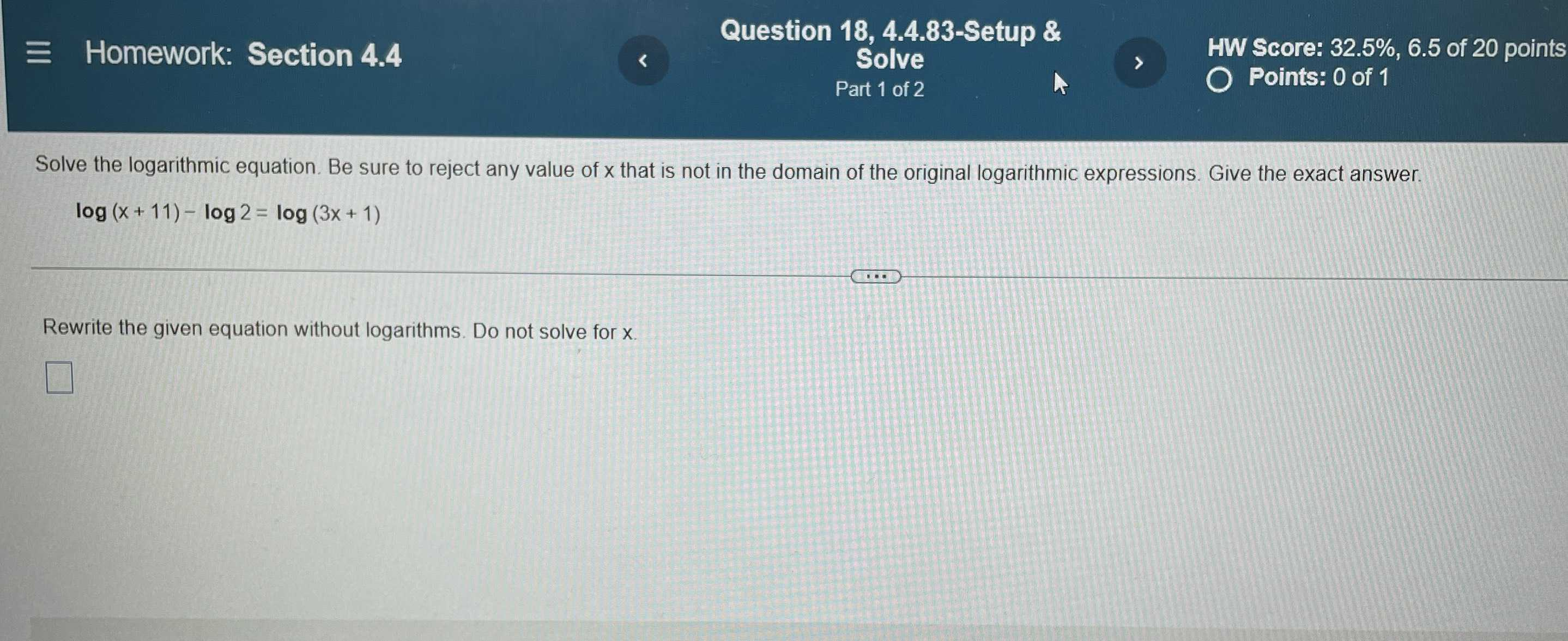### Still have math questions?

Algebra
QuestionSolve the logarithmic equation. Be sure to reject any value of $$x$$ that is not in the domain of the original logarithmic expressions. Give the exact answer.

$$\log ( x + 11 ) - \log 2 = \log ( 3 x + 1 )$$

Rewrite the given equation without logarithms. Do not solve for $$x$$

$$\frac{x+ 11}{2}= 3x+ 1$$